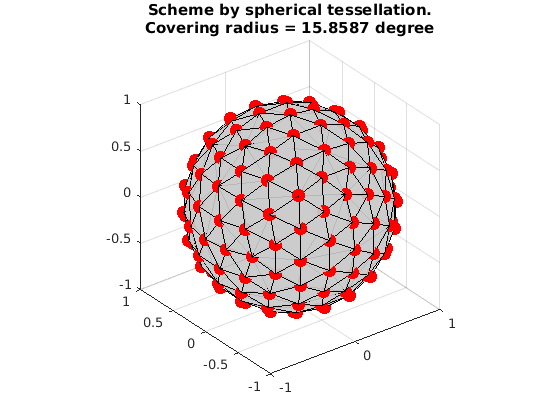# Generate a single shell scheme by using IMOC + 1-Opt + CNLO

This is a demo to generate a single-shell scheme with 81 samples by using IMOC + 1-Opt + CNLO.

OptimalSamplingSingleShellCNLO is in matlab. But to run IMOC and 1-Opt, you need to build the dmritool C++ codes.

Reference:

1. "Single- and Multiple-Shell Uniform Sampling Schemes for Diffusion MRI Using Spherical Codes", Jian Cheng, Dinggang Shen, Pew-Thian Yap, Peter J. Basser, IEEE Transactions on Medical Imaging, 2017.

2. "Novel single and multiple shell uniform sampling schemes for diffusion MRI using spherical codes", Jian Cheng, Dinggang Shen, Pew-Thian Yap, Peter J. Basser, MICCAI 2015.

Copyright (c) 2016, Jian Cheng (jian.cheng.1983@gmail.com)

## IMOC to design a single shell scheme (81 samples)

```tic
! SamplingSchemeQSpaceIMOCEstimation grad_81_IMOC.txt --tessOrder 7 --numberOfSamples 81
toc

grad_IMOC = ReadDirections('grad_81_IMOC_shell1.txt');

fprintf('\nConvering radius:\t %10.3f\n',CoveringRadius(grad_IMOC)*180/pi);

VisualizeMultiShellScheme(grad_IMOC);
title({'Scheme by IMOC.', ['Covering radius = ', num2str(CoveringRadius(grad_IMOC)*180/pi), ' degree']});
```
```Elapsed time : 9.17745
save orientations in shell 1 to grad_81_IMOC_shell1.txt
Elapsed time is 9.321357 seconds.

Convering radius:	     15.740
```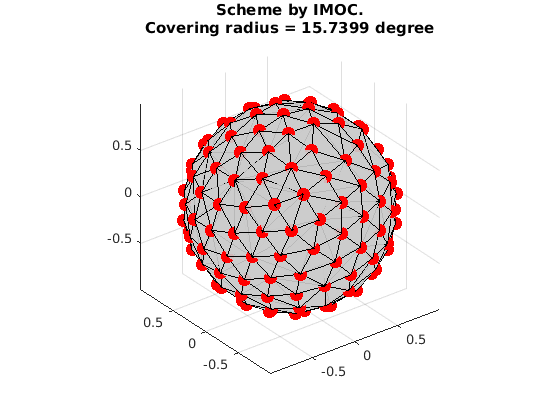## IMOC + 1-Opt to design a single shell scheme (81 samples)

```tic
! SamplingSchemeQSpace1OptEstimation grad_81_IMOC1Opt.txt --initial grad_81_IMOC_shell1.txt --tessOrder 7
toc

grad_IMOC1Opt = ReadDirections('grad_81_IMOC_shell1.txt');

fprintf('\nConvering radius:\t %10.3f\n',CoveringRadius(grad_IMOC1Opt)*180/pi);

VisualizeMultiShellScheme(grad_IMOC1Opt);
title({'Scheme by IMOC + 1-Opt.', ['Covering radius = ', num2str(CoveringRadius(grad_IMOC1Opt)*180/pi), ' degree']});
```
```Elapsed time : 3.21282
save orientations in shell 1 to grad_81_IMOC1Opt_shell1.txt
Elapsed time is 3.335626 seconds.

Convering radius:	     15.740
```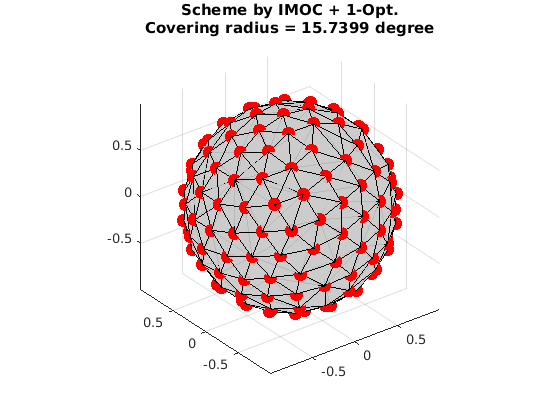## IMOC + 1-Opt + CNLO to design a single shell scheme (81 samples)

```clear param;

% weight between individual shell and combined shell
param.w  = 0.5;

% maxtime for running.
param.maxtime = 600;
param.verbose = 1;

tic
[grad_IMOC1Opt_CNLO,xopt, fopt, retcode] = OptimalSamplingSingleShellCNLO(grad_IMOC1Opt, param);
toc

fprintf('\nConvering radius:\t %10.3f\n',CoveringRadius(grad_IMOC1Opt_CNLO)*180/pi);

VisualizeMultiShellScheme(grad_IMOC1Opt_CNLO);
title({'Scheme by IMOC + 1Opt + CNLO.', ['Covering radius = ', num2str(CoveringRadius(grad_IMOC1Opt_CNLO)*180/pi), ' degree']});
```
```Elapsed time is 43.513162 seconds.

Convering radius:	     16.506
```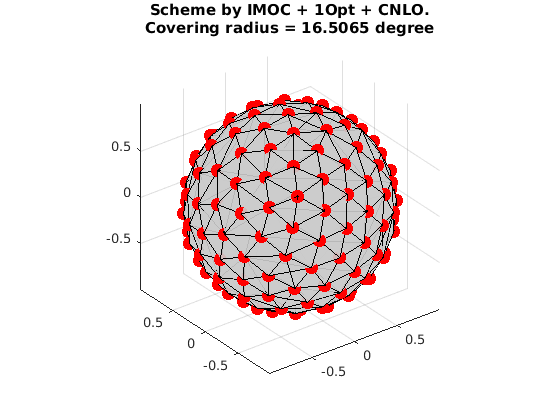## Compared with the single shell scheme with 81 samples by EEM in CAMINO

```grad_EEM = ReadDirections([getenv('HOME'), '/.dmritool/Data/ElectricRepulsion/Elec081.txt']);

fprintf('\nConvering radius:\t %10.3f\n',CoveringRadius(grad_EEM)*180/pi);

VisualizeMultiShellScheme(grad_EEM);
title({'Scheme by EEM.', ['Covering radius = ', num2str(CoveringRadius(grad_EEM)*180/pi), ' degree']});
```
```Convering radius:	     15.711
```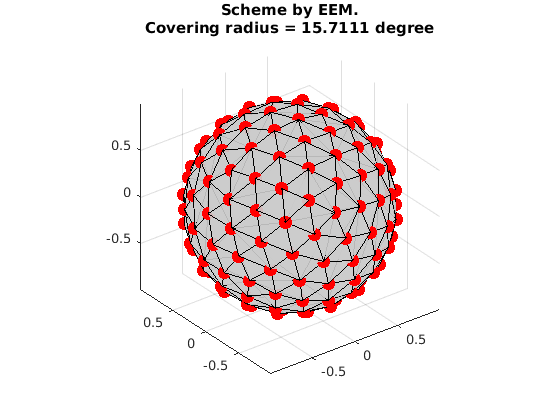## Compared with the single shell scheme with 81 samples by spherical tessellation

```grad_Tess = ReadDirections([getenv('HOME'), '/.dmritool/Data/Tessellation/directions_t3.txt']);

fprintf('\nConvering radius:\t %10.3f\n',CoveringRadius(grad_Tess)*180/pi);

VisualizeMultiShellScheme(grad_Tess);
title({'Scheme by spherical tessellation.', ['Covering radius = ', num2str(CoveringRadius(grad_Tess)*180/pi), ' degree']});
```
```Convering radius:	     15.859
```